# Determine the functional regulations assignment

The picture shows a radar dish.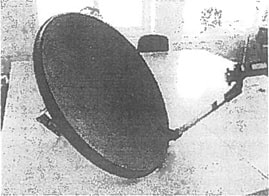Figure 4 shows the graph of the function f, which describes the curve that appears in a section through the dish. The function f is given by the calculation regulations:

f(x)=5,30*10-3, where .x € [-35:35]

All measurements are in cm.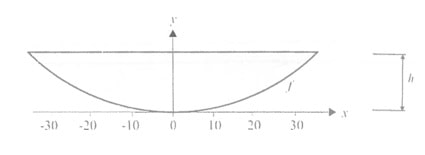1. Determine the height h as shown in figure 4.

The line t is tangent to the graph of f at the point A. Point A has the coordinates (20; f (20)).

1. Determine the slope of the tangent
2. Set the equation of the key for t.

If a coordinate system is inserted in a section along the badminton court so that the center of the badminton court is in (0; 0), one can describe the ball's path by a long serve, by the following function, where the y-coordinate indicates the height of the ball above the floor measured in meters.

y=f(x)=-4,293*10-3*x4- 4,386*10-3 x3 - 3,790*10-3 x2 +0,9784x+ 3,468

where x>-2,5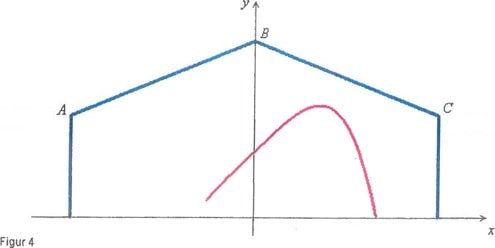t the inlaid coordinate system seen in Figure 4, the serving badminton player strikes the ball where x = -2.5.

1. To what height does the badminton player hit the badminton ball?
1. Determine the x-coordinate of the place where the badminton ball lands after the serve.
2. Determine the maximum height that the badminton ball achieves in the long serve.

An exponential development f (t) = 5 * 1.2t, t is this year

1. How many percent grow f (t) per year?
2. What value does the doubling time have?
3. c) What year has f (t) reached the value 1200?

An exponential development goes through the two points (1; 4) and (5; 28)

1. Set up an equation system to determine the function regulation.
2. Determine the functional regulations.

Throughout history, gold rings for men have been popular among kings. regents and army leaders Within the aka world there is a doctor ring in Denmark and Sweden that can be worn by people. Having acquired a doctorate time, it has become more common for men to wear rings. These are often made of gold, white gold or platinum and often with different types of stones.

The picture shows a gold ring with the gemstones onyx (black) and a diamond.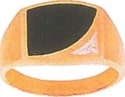In Figure 1, the onyx is seen as a tinted area in a coordinate system. The area is bounded by the y-axis as well as the graph functions f and g with the regulations.

f(x) = - 0,033x4 + 0,10x3- 0,26x2 + 0,29x + k

g(x) = 0,32x2,6

All dimensions are in centimeters. The value of k was determined in question 1a).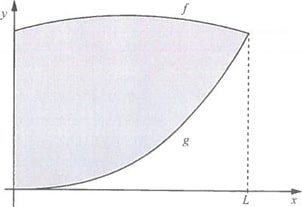1. Determine the value of L indicating the length of the pitch range, see figure 1.

The height is defined as the vertical distance between the graphs of f and g.

1. Determine the height when x = l.
2. Set a rule for the function h that specifies the height of any x: 0 <x <L.
3. Determine the maximum height of the onyx area.

The piece of onyx used for the ring is 0,1 cm thick everywhere.

1. Determine the volume of the onyx piece.